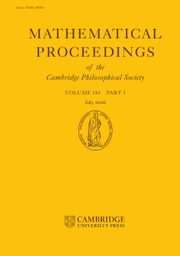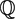Home
Hostname: page-component-5c569c448b-ph4cd Total loading time: 0.445 Render date: 2022-07-03T16:53:33.022Z Has data issue: true Feature Flags: { "shouldUseShareProductTool": true, "shouldUseHypothesis": true, "isUnsiloEnabled": true, "useRatesEcommerce": false, "useNewApi": true } hasContentIssue trueMathematical Proceedings of the Cambridge Philosophical Society

# Low-lying zeros of elliptic curve L-functions: Beyond the Ratios Conjecture

Published online by Cambridge University Press:  08 January 2016

## Abstract

We study the low-lying zeros of L-functions attached to quadratic twists of a given elliptic curve E defined over$\mathbb{Q}$. We are primarily interested in the family of all twists coprime to the conductor of E and compute a very precise expression for the corresponding 1-level density. In particular, for test functions whose Fourier transforms have sufficiently restricted support, we are able to compute the 1-level density up to an error term that is significantly sharper than the square-root error term predicted by the L-functions Ratios Conjecture.

Type
Research Article
Information

## Access options

Get access to the full version of this content by using one of the access options below. (Log in options will check for institutional or personal access. Content may require purchase if you do not have access.)

## References

[BCDT]Breuil, C., Conrad, B., Diamond, F. and Taylor, R.On the modularity of elliptic curves over Q: wild 3-adic exercises. J. Amer. Math. Soc. 14 (2001), no. 4, 843939.CrossRefGoogle Scholar
[B]Brumer, A.The average rank of elliptic curves I. Invent. Math. 109 (1992), no. 3, 445472.CrossRefGoogle Scholar
[CFZ]Conrey, J. B., Farmer, D. W. and Zirnbauer, M. R.Autocorrelation of ratios of L-functions. Commun. Number Theory Phys. 2 (2008), no. 3, 593636.CrossRefGoogle Scholar
[CS]Conrey, J. B. and Snaith, N. C.Applications of the L-functions ratios conjectures. Proc. Lond. Math. Soc. (3) 94 (2007), no. 3, 594646.CrossRefGoogle Scholar
[C]Cremona, J. E.Algorithms for modular elliptic curves, second edition. (Cambridge University Press, Cambridge, 1997).Google Scholar
[D]Davenport, H.Multiplicative Number Theory, third edition. Revised and with a preface by H. L. Montgomery. Graduate Texts in Mathematics 74. (Springer-Verlag, New York, 2000).Google Scholar
[FM]Fiorilli, D. and Miller, S. J.Surpassing the ratios conjecture in the 1-level density of Dirichlet L-functions. Algebra Number Theory 9 (2015), no. 1, 1352.CrossRefGoogle Scholar
[G]Goldfeld, D.Conjectures on elliptic curves over quadratic fields. Number Theory, Carbondale 1979 (Proc. Southern Illinois Conf., Southern Illinois Univ., Carbondale, Ill., 1979), pp. 108118, Lecture Notes in Math. 751. (Springer, Berlin, 1979).Google Scholar
[H-B]Heath-Brown, D. R.The average analytic rank of elliptic curves. Duke Math. J. 122 (2004), no. 3, 591623.CrossRefGoogle Scholar
[H]Hejhal, D. A.On the triple correlation of zeros of the zeta function. Internat. Math. Res. Notices (1994), no. 7, 293302.CrossRefGoogle Scholar
[HKS]Huynh, D. K., Keating, J. P. and Snaith, N. C.Lower order terms for the one-level density of elliptic curve L-functions. J. Number Theory 129 (2009), no. 12, 28832902.CrossRefGoogle Scholar
[HMM]Huynh, D. K., Miller, S. J. and Morrison, R.An elliptic curve test of the L-functions ratios conjecture. J. Number Theory 131 (2011), no. 6, 11171147.CrossRefGoogle Scholar
[I]Iwaniec, H.On the order of vanishing of modular L-functions at the critical point. Sém. Théor. Nombres Bordeaux (2) 2 (1990), no. 2, 365376.CrossRefGoogle Scholar
[IK]Iwaniec, H. and Kowalski, E.Analytic Number Theory, Amer. Math. Soc. Colloq. Publ. 53. (American Mathematical Society, Providence, RI, 2004).CrossRefGoogle Scholar
[KaS1]Katz, N. M. and Sarnak, P. Zeros of zeta functions, their spacings and their spectral nature, preprint (1997).Google Scholar
[KaS2]Katz, N. M. and Sarnak, P.Zeroes of zeta functions and symmetry. Bull. Amer. Math. Soc. (N.S.) 36 (1999), no. 1, 126.CrossRefGoogle Scholar
[KaS3]Katz, N. M. and Sarnak, P.Random Matrices, Frobenius Eigenvalues and Monodromy. Amer. Math. Soc. Colloq. Publ. 45. (American Mathematical Society, Providence, RI, 1999).Google Scholar
[KeS1]Keating, J. P. and Snaith, N. C.Random matrix theory and ζ(1/2 + it). Comm. Math. Phys. 214 (2000), no. 1, 5789.CrossRefGoogle Scholar
[KeS2]Keating, J. P. and Snaith, N. C.Random matrix theory and L-functions at s = 1/2. Comm. Math. Phys. 214 (2000), no. 1, 91110.CrossRefGoogle Scholar
[Me]Mestre, J.-F.Formules explirefs et minorations de conducteurs de variétés algébriques. Compositio Math. 58 (1986), no. 2, 209232.Google Scholar
[Mi]Michel, P.Rang moyen de familles de courbes elliptiques et lois de Sato–Tate. Monatsh. Math. 120 (1995), no. 2, 127136.CrossRefGoogle Scholar
[Mo]Montgomery, H. L.The pair correlation of zeros of the zeta function. Analytic Number Theory (Proc. Sympos. Pure Math., Vol. XXIV, St. Louis Univ., St. Louis, Mo., 1972) (Amer. Math. Soc., Providence, RI, 1973) pp. 181193.CrossRefGoogle Scholar
[MV]Montgomery, H. L. and Vaughan, R. C.Multiplicative Number Theory. I. Classical theory. Cambridge Studies in Advanced Mathematics 97 (Cambridge University Press, Cambridge, 2007).Google Scholar
[O1]Odlyzko, A. M.On the distribution of spacings between zeros of the zeta function. Math. Comp. 48 (1987), no. 177, 273308.CrossRefGoogle Scholar
[O2]Odlyzko, A. M. The 1020th zero of the Riemann zeta function and 70 million of its neighbours, preprint, (1989). Available on http://www.dtc.umn.edu/~odlyzko/unpublished/index.html.Google Scholar
[RS1]Rudnick, Z. and Sarnak, P.The n-level correlations of zeros of the zeta function. C. R. Acad. Sci. Paris Sér. I Math. 319 (1994), no. 10, 10271032.Google Scholar
[RS2]Rudnick, Z. and Sarnak, P.Zeros of principal L-functions and random matrix theory. Duke Math. J. 81 (1996), no. 2, 269322.CrossRefGoogle Scholar
[Sh]Shimura, G.On the holomorphy of certain Dirichlet series. Proc. London Math. Soc. (3) 31 (1975), no. 1, 7998.CrossRefGoogle Scholar
[Si]Silverman, J. H.The Arithmetic of Elliptic Curves, second edition. Graduate Texts in Mathematics 106. (Springer, Dordrecht, 2009).CrossRefGoogle Scholar
[TW]Taylor, R. and Wiles, A.Ring-theoretic properties of certain Hecke algebras. Ann. of Math. (2) 141 (1995), no. 3, 553572.CrossRefGoogle Scholar
[W]Wiles, A.Modular elliptic curves and Fermat's last theorem. Ann. of Math. (2) 141 (1995), no. 3, 443551.CrossRefGoogle Scholar
[Y1]Young, M. P.Lower-order terms of the 1-level density of families of elliptic curves. Int. Math. Res. Not. (2005), no. 10, 587633.CrossRefGoogle Scholar
[Y2]Young, M. P.Low-lying zeros of families of elliptic curves. J. Amer. Math. Soc. 19 (2006), no. 1, 205250.CrossRefGoogle Scholar
7
Cited by

# Save article to Kindle

Note you can select to save to either the @free.kindle.com or @kindle.com variations. ‘@free.kindle.com’ emails are free but can only be saved to your device when it is connected to wi-fi. ‘@kindle.com’ emails can be delivered even when you are not connected to wi-fi, but note that service fees apply.

Find out more about the Kindle Personal Document Service.

Low-lying zeros of elliptic curve L-functions: Beyond the Ratios Conjecture
Available formats
×

# Save article to Dropbox

To save this article to your Dropbox account, please select one or more formats and confirm that you agree to abide by our usage policies. If this is the first time you used this feature, you will be asked to authorise Cambridge Core to connect with your Dropbox account. Find out more about saving content to Dropbox.

Low-lying zeros of elliptic curve L-functions: Beyond the Ratios Conjecture
Available formats
×

# Save article to Google Drive

To save this article to your Google Drive account, please select one or more formats and confirm that you agree to abide by our usage policies. If this is the first time you used this feature, you will be asked to authorise Cambridge Core to connect with your Google Drive account. Find out more about saving content to Google Drive.

Low-lying zeros of elliptic curve L-functions: Beyond the Ratios Conjecture
Available formats
×
×